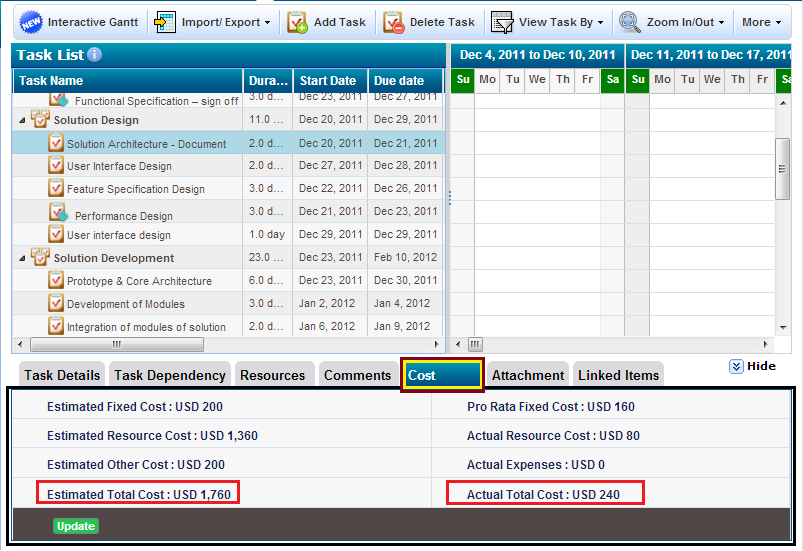Track project cost at Task Level

Project owner and senior managers up in the hierarchy can track project cost at task level as shown below.

The costs you can track at project level are (i) Estimated cost (ii) Actual costEstimated Total Cost = Estimated fixed cost + Estimated resource Cost (assigned tasks, resource rates) + Estimated other cost

 Actual Total Cost = Fixed cost (pro-rata, based on % completion of task) + Actual resource cost (approved timesheet entries) + Actual expense (approved expense sheets)

Let's understand what each of these teams mean

Estimated Fixed cost: When project planner expects to incur some fixed cost in order to complete a given task, it will be added to Estimated Fixed Cost element. It can be equipment cost, some fixed consultation charges, etc that a project has to incur in order to carry out that task. Project manager can enter Estimated Fixed Cost by clicking on Update button available in the bottom section of Cost as shown above.

Estimated Resource Cost: When project manager add any resource to a project team as a team member, s/he specifies applicable cost rate (per hour), overtime rate (per hour) and billing rate (per hour). And as project manager starts assigning tasks to a given resource, s/he is assigned certain hours of effort for those tasks. Thus each resource is expected to cost a project specified hours of work multiplied by his/her cost rate (per hour). Thus Estimated Resource Cost at a task level is is summation of all resources cost based on task level efforts (hours) assigned to resource(s) and their applicable cost rate.

Estimated Other Cost: Beside Estimated Fixed Cost and Estimated Resource Cost, any other cost that a project manager is expecting to incur in order to complete a given task will be considered as Estimated Other cost. Project manager can enter Estimated Other Cost by clicking on Update button available in the bottom section of the Cost as shown above.

Actual Fixed Cost: As task is being executed/progressed, depending on the percentage completion level; the actual fixed cost is proportionately calculated from Estimated Fixed Cost e.g. Estimated Fixed Cost is USD 100 and task progress is 20%, then Actual Fixed Cost is USD 20; when the task progress will be updated to say 75%, the Actual Fixed Cost will be USD 75 and so on. Essentially, Actual Fixed Cost is pro-rated from Estimated Fixed Cost and percentage completion of task.

Actual Resource Cost: As team members start entering timesheet hours against project tasks assigned to them, and as project manager approves those hours, it becomes actual resource-work hours against a given task. In order to calculate Actual Resource Cost, ZilicusPM system uses project specific resource cost rate (per hour) and overtime cost rate (per hour) multiplied by approved timesheet hours for a given resources.

So for a given resource actual resource cost for a given task will be = approved regular timesheet hours * cost rate (per hour) + approved overtime timesheet hours * overtime cost rate (per hour)

Sum of individual resource cost for team members working on a given task and their applicable cost rate, multiplied by approved timesheet hours will give Actual Resource Cost for a given task.

Actual Expenses: When project team member incurs expenses (like travel, stay, lunch/food) while completing/progressing a given task, s/he can enter those expenses against project task in Zilicus. All such expenses incurred against a given task, by all applicable resources will be put together to give Actual Expenses.

Note: Project team members, can NOT view project budget, cost and billing information, including resource rate details. Only if a project team member is given a privilege to modify schedule, s/he can track cost element at task level (but without knowing specific resource's cost/billing rate per hour).